# Volume - math word problems

1. Tank diameterA cylindrical tank has a volume of 60 hectoliters and is 2.5 meters deep. Calculate the tank diameter.
2. Pool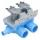Pool is filled with two water supply. The first supply fill pool for nine hours, the second for six hours. How many hours will take fill the pool when the water flows in through the first supply 3 hours and then we will open a second supply?
3. Cone containerRotary cone-shaped container has a volume 1000 cubic cm and a height 12 cm. Calculate how much metal we need for making this package.
4. Bottles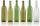Mrs. Violet brewed 15 l of raspberry juice. She poured it into 17 bottles. Some were 3/4 liter and some one liter. How many was bottles of this two types?
5. Gravel - cone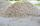Mound of gravel has shape of regular circular cone with a height 3.3 meter and a base circumference of 18.85 meters. How many cubic meters of gravel are in the pile? Calculate the weight of gravel if its density is p = 640 kg / cubic m.
6. Truncated coneA truncated cone has a bases radiuses 40 cm and 10 cm and a height of 25 cm. Calculate its surface area and volume.
7. PrismThe base of a perpendicular triangular prism is a right triangle with legs 4.5 cm and 6 cm long. What is the surface of the prism, if its volume is 54 cubic centimeters?
8. Triangular prismBase of perpendicular triangular prism is a right triangle with leg length 5 cm. Content area of the largest side wall of its surface is 130 cm² and the height of the body is 10 cm. Calculate its volume.
9. Volume of three cuboids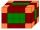Calculate the total volume of all cuboids for which the the size of the edges are in a ratio of 1:2:3, and one of the edges has a size 6 cm.
10. Bricks wallThere are 5000 bricks. How high wall thickness of 20 cm around the area which has dimensions 20 m and 15 m can use these bricks to build? Brick dimensions are 30 cm, 20 cm and 10 cm.
11. Water level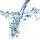To cuoid shaped poll, bottom size 2m and 3.5m; flows water at a rate of 50 liters per minute. How long will take to water reach level 50 cm?
12. Iron cast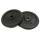What is the weight of cast iron with volume 3575 cubic centimeters. The density of the cast iron is 7600 kg/m3
13. Excavator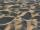The excavator bucket capacity is 0.5 m3. Determine the mass of sand that the excavator picks up. The sand density is 1650 kg/m3.
14. PrismFind the volume and surface area of prism with base of an equilateral triangle with side 7 dm long and the body height of 1.5 m.
15. Wood cuboidWhat is the weight of the wood cuboid 15 cm, 20 cm, 3 m if 1 m3 wood weighs 800 kg?
16. Decagon prism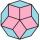A regular decagon of side a = 2 cm is the base of the perpendicular prism, the side walls are squares. Find the prism volume in cm3, round to two decimal places.
17. RingThe ring made from gold and copper alloy has a weight of 14.5 g and a volume of 1.03 cm3. How much gold and how much copper it contains? The metal densities are Au 19.3 g/cm³ and Cu 8.94 kg·dm-3
18. Hexa prismDetermine the volume of hex prism with edge base 4 cm. The body height is 28 cm.
19. FreezerThe freezer has the shape of a cuboid with internal dimensions of 12 cm, 10 cm, 30 cm. A layer of ice of 23 mm thick was formed on the inner walls (and on the opening) of the freezer. How many liters of water will drain if we dispose the freezer?
20. Prism - eq triangleCalculate the volume and surface of the prism with the base of an equilateral triangle with side a = 4cm and the body height is 6cm.

Do you have an interesting mathematical word problem that you can't solve it? Submit math problem, and we can try to solve it.

We will send a solution to your e-mail address. Solved examples are also published here. Please enter the e-mail correctly and check whether you don't have a full mailbox.

Please do not submit problems from current active competitions such as Mathematical Olympiad, correspondence seminars etc...